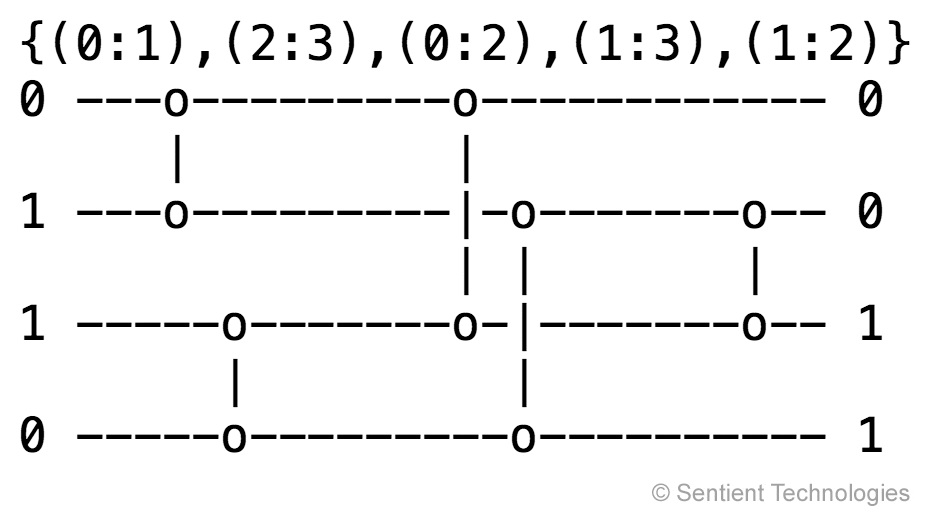# How Composite Novelty Overcomes Deception and Discovers Minimal Sorting Networks

This demo shows how the composite novelty method of Paper 1 discovers an optimal solution to the 8-line sorting network problem, while the standard multiobjective optimization method NSGA-II gets stuck in a suboptimal solution.A sorting network is a hardware design where a set of numbers are given on separate input lines and the network gives these numbers in a sorted order in separate output lines. The sorting is performed by several comparators that each compare the numbers in their two input lines and swap them if they are in an incorrect order. The comparators can be grouped into layers where they operate in parallel (layers are separated by a wider gap between comparators above). The goal is to find a network that sorts correctly with as few layers as possible (making the sorting fast) and as few comparators as possible (reducing hardware requirements). As an example, an optimal 4-line sorting network is given above: it consists of five comparators, grouped into three layers. With the given input, the comparators at bottom left and at right swap their inputs and the other three leave them as is. Note that in order to verify that a sorting network is correct, it is only necessary to test it on all binary inputs (Knuth 1998).

Minimal sorting networks are theoretically known for up to 8 input lines. Finding smaller networks for larger sizes is an interesting optimization problem, and evolutionary computation is well suited for it: many of the known minimal networks with more than 8 lines have been discovered through evolution. The problem is difficult because it is deceptive: in order to minimize a network further, it may be necessary to change it drastically, i.e. to make it worse before it can become better. Thus, sorting network minimization is a good problem for demonstrating the power of composite novelty in solving hard problems.

In the demo, NSGA-II and composite novelty run in parallel on the 8-line problem. Each frame shows the current best network found by each generation, based on correctness first, then number of layers, then number of comparators. The comparators are specified numerically above. The line below shows the behavioral characterization in blue (i.e. number of swaps in each line), the correctness in red (i.e. the sum of edit distances over the 256 inputs), the number of layers in orange, and the number of comparators in green. Play the video to see how these methods perform!

As you can see in the animation, both methods start from scratch, and first have to grow the network to make it correct (which is the primary dimension of fitness). Once they find correct networks, they start minimizing them. After a while, NSGA-II finds a network with 8 layers and 22 comparators, but is unable to improve upon it. In contrast, composite novelty improves the network several times after this critical point. Why? The reason is evident in the search-space demo: The candidates in the composite novelty population represent useful tradeoffs between objectives, and they map to much more diverse behaviors. As a result, the composite novelty method is able to find tradeoffs between the objectives that allow it to get around the deceptive area of the landscape. It will then eventually find drastically different designs, including an optimal network with 6 layers and 19 comparators.